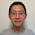## Sunday, June 17, 2012

### HSL - RGB Conversion Algorithms in C

1.RGB To HSL Conversion
This function will create a HSL model from a RGB model. The algorithm behind the conversion is described by the formulas below.RGB to HSL algorithm
The meaning of the variables:
M - the RGB component with the greatest value
m - the RGB component with the smalles value
c - chroma
r,g,b - the components of the RGB model (red, green, blue)
h,s,l - the components of HSL model (hue, saturation, lightness)

The components of the RGB model (r,g,b), saturation (s)  and intensity (i) should have values in the range [0,1], while the hue (h) should have values in the rnage [0,360].

The prototype of the function is:
```/* Description:
*  Creates a HslColor structure from RGB components
* Parameters:
*  r,g,b - the components of an RGB model expressed
*          as real numbers
* Returns:
*  A pointer to the HslColor is the parameters are
* correct. Otherwise returns NULL.
*/
HslColor* Hsl_CreateFromRgbF(double r, double g, double b);```
The function can be implemented as:
```HslColor* Hsl_CreateFromRgbF(double r, double g, double b)
{
double M = 0.0, m = 0.0, c = 0.0;
HslColor* color = NULL;
if (RgbF_IsValid(r, g, b) == true)
{
M = Double_GetMaximum(r, g, b);
m = Double_GetMinimum(r, g, b);
c = M - m;
color = Hsl_Create(0.0, 0.0, 0.0);
color->L = 0.5 * (M + m);
if (c != 0.0)
{
if (M == r)
{
color->H = fmod(((g - b) / c), 6.0);
}
else if (M == g)
{
color->H = ((b - r) / c) + 2.0;
}
else/*if(M==b)*/
{
color->H = ((r - g) / c) + 4.0;
}
color->H *= 60.0;
color->S = c / (1.0 - fabs(2.0 * color->L - 1.0));
}
}
return color;
}```
2.HSL to RGB Conversion
This function will create a RGB model from an HSL model. The algorithm behind the conversion is described by the formulas below.HSL to RGB algorithm
The meaning of the variables:
c - chroma
x - intermediate value used for computing r,g,b
m - intermediate value used for creating the r,g,b baseline
r,g,b - the components of the RGB model (red, green, blue)
h,s,l - the components of HSL model (hue, saturation, lightness)

The components of the RGB model (r,g,b), saturation (s)  and intensity (i) should have values in the range [0,1], while the hue (h) should have values in the rnage [0,360].

The prototype of the function is:
```/*
* Description:
*  Creates a RgbFColor structure from HSL components
* Parameters:
*  h,s,l - the components of an HSL model expressed
*          as real numbers
* Returns:
*  A pointer to the RgbFColor is the parameters are
* correct. Otherwise returns NULL.
*/
RgbFColor* RgbF_CreateFromHsl(double h, double s, double l);```
The function can be implemented as:
```RgbFColor* RgbF_CreateFromHsl(double h, double s, double l)
{
RgbFColor* color = NULL;
double c = 0.0, m = 0.0, x = 0.0;
if (Hsl_IsValid(h, s, l) == true)
{
c = (1.0 - fabs(2 * l - 1.0)) * s;
m = 1.0 * (l - 0.5 * c);
x = c * (1.0 - fabs(fmod(h / 60.0, 2) - 1.0));
if (h >= 0.0 && h < (HUE_UPPER_LIMIT / 6.0))
{
color = RgbF_Create(c + m, x + m, m);
}
else if (h >= (HUE_UPPER_LIMIT / 6.0) && h < (HUE_UPPER_LIMIT / 3.0))
{
color = RgbF_Create(x + m, c + m, m);
}
else if (h < (HUE_UPPER_LIMIT / 3.0) && h < (HUE_UPPER_LIMIT / 2.0))
{
color = RgbF_Create(m, c + m, x + m);
}
else if (h >= (HUE_UPPER_LIMIT / 2.0)
&& h < (2.0f * HUE_UPPER_LIMIT / 3.0))
{
color = RgbF_Create(m, x + m, c + m);
}
else if (h >= (2.0 * HUE_UPPER_LIMIT / 3.0)
&& h < (5.0 * HUE_UPPER_LIMIT / 6.0))
{
color = RgbF_Create(x + m, m, c + m);
}
else if (h >= (5.0 * HUE_UPPER_LIMIT / 6.0) && h < HUE_UPPER_LIMIT)
{
color = RgbF_Create(c + m, m, x + m);
}
else
{
color = RgbF_Create(m, m, m);
}
}
return color;
}```
3.Example
```#include<stdio.h>
#include"colorspace.h"

int main(int argc, char** argv)
{
HslColor* hsl = NULL;
RgbFColor* rgbF = NULL;
RgbIColor* rgbI = NULL;

/*HSI to RGB*/
hsl = Hsl_Create(84.0, 1.0, 0.4);
rgbF = RgbF_CreateFromHsl(hsl->H, hsl->S, hsl->L);
rgbI = RgbI_CreateFromRealForm(rgbF->R, rgbF->G, rgbF->B);
printf("\nHSL : %f %f %f", hsl->H, hsl->S, hsl->L);
printf("\nRGBf : %f %f %f", rgbF->R, rgbF->G, rgbF->B);
printf("\nRGBi : %d %d %d", rgbI->R, rgbI->G, rgbI->B);

/*Frees the resources*/
free(hsl);
free(rgbF);
free(rgbI);

/*RGB to HSI*/
rgbI = RgbI_Create(108U, 198U, 78U);
rgbF = RgbF_CreateFromIntegerForm(rgbI->R, rgbI->G, rgbI->B);
hsl = Hsl_CreateFromRgbF(rgbF->R, rgbF->G, rgbF->B);
printf("\nHSL : %f %f %f", hsl->H, hsl->S, hsl->L);
printf("\nRGBf : %f %f %f", rgbF->R, rgbF->G, rgbF->B);
printf("\nRGBi : %d %d %d", rgbI->R, rgbI->G, rgbI->B);
return 0;
}
/*
HSL : 84.000000 1.000000 0.400000
RGBf : 0.480000 0.800000 0.000000
RGBi : 122 204 0
HSL : 105.000000 0.512821 0.541176
RGBf : 0.423529 0.776471 0.305882
RGBi : 108 198 78
*/```

1.Great article ~ ~

Report a bug:

In figure "RGB to HSL algorithm", "b = m" should be "b = M" :)

1.